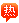##用户名 Email 自动登录 找回密码 密码 立即注册# 林培伟：3道应用题解答一下

2020-8-12 17:29| 发布者: admin| 查看: 137| 评论: 0

### 热I2的回答：

1.长加宽：36÷2=18     18÷（7+2）=2    长：2×7=14（cm）    实际：14×500÷100==70（m）    宽：2×2=4  （cm）    实际：4×500÷100=20（m） 2.化简比例尺：          1：8000000    两地距离：8.4×8000000=67200000（cm）=672（km）    时间：672÷64=10.5（h） 3.男生：42×4/7=24（人）    女士：24÷6=4     4×5=20（人） 好了，纯手工！楼上最后一题错了！！ 这题目小学的吧..

### 热I2的回答：

1.长加宽：36÷2=18     18÷（7+2）=2    长：2×7=14（cm）    实际：14×500÷100==70（m）    宽：2×2=4  （cm）    实际：4×500÷100=20（m） 2.化简比例尺：          1：8000000    两地距离：8.4×8000000=67200000（cm）=672（km）    时间：672÷64=10.5（h） 3.男生：42×4/7=24（人）    女士：24÷6=4     4×5=20（人） 好了，纯手工！楼上最后一题错了！！ 这题目小学的吧..

### 披披的回答：

1

1、长宽之和：36÷2=18（cm） 2、长：18÷（7＋2）×7=14（cm） 3、宽：18－14=4（cm） 4、实际长：14÷1/500=7000（cm）=70（m） 5、实际宽：4÷1/500=2000（cm）=20（m）长是（70）米，宽是（20）米 28.4*80=672 千米672÷64=10.5小时342×4/7=24 42-24=18人 现在全班女生有18人

### 最新评论

GMT+8, 2022-7-2 18:45 , Processed in 0.078376 second(s), 28 queries .